# extremeStat: quantile estimation

#### 2023-08-29

Vignette Rmd source

The R package extremeStat, available at github.com/brry, contains code to fit, plot and compare several (extreme value) distribution functions. It can also compute (truncated) distribution quantile estimates and draw a plot with return periods on a linear scale.

Main focus of this document:
Quantile estimation via distribution fitting
Comparison of GPD implementations in several R packages
Extreme Value Statistics

Note: in some disciplines, quantiles are called percentiles, but technically, percentiles are only one kind of quantiles (as are deciles, quartiles, etc).

## Motivational example

library(extremeStat)
data("annMax")
extremes <- distLextreme(annMax, RPs=c(2,5,10,20,50,100,200), gpd=FALSE)
plotLextreme(extremes, log="x", xlim=c(1,200), ylim=c(35,140), nbest=7)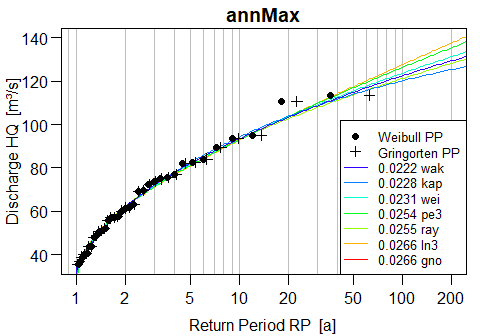head(extremes$returnlev) ## RP.2 RP.5 RP.10 RP.20 RP.50 RP.100 RP.200 ## wak 62.06908 82.00224 93.37393 103.3017 114.8184 122.5049 129.4158 ## kap 61.63990 82.43319 94.20990 103.8075 113.9858 120.1919 125.3644 ## wei 61.84405 81.72957 93.39678 103.5552 115.4695 123.6596 131.3094 ## pe3 61.86107 81.13112 92.97753 103.7200 116.8781 126.2917 135.3646 ## ray 62.37416 81.92136 93.07332 102.6385 113.7131 121.2474 128.2329 ## ln3 61.89473 80.85126 92.71419 103.6903 117.4749 127.5938 137.5657 More on extreme value statistics in the last section. TOC ## Package installation install.packages("extremeStat") # install the development version on github, incl. vignette: if(!requireNamespace("remotes", quitly=TRUE)) install.packages("remotes") remotes::install_github("brry/extremeStat", build_vignettes=TRUE) extremeStat has 33 dependencies, because of the GPD comparison across the packages. library(extremeStat) TOC ## Example dataset Let’s use the dataset rain with 17k values. With very small values removed, as those might be considered uncertain records, this leaves us with 6k values. data(rain, package="ismev") rain <- rain[rain>2] hist(rain, breaks=80, col=4, las=1) # Visual inspection is easier on a logarithmic scale: berryFunctions::logHist(rain, breaks=80, col=3, las=1)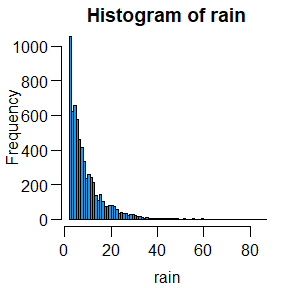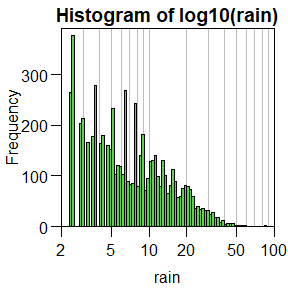TOC ## Fitting distributions The function distLfit fits 17 of the distribution types avalable in the R package lmomco (there are more, but some of these require quite a bit of computation time and are prone to not be able to be fitted to this type of data distribution anyways. Turn them on with speed=FALSE). The parameters are estimated via L-moments. These are analogous to the conventional statistical moments (mean, variance, skewness and kurtosis), but “robust [and] suitable for analysis of rare events of non-Normal data. L-moments are consistent and often have smaller sampling variances than maximum likelihood in small to moderate sample sizes. L-moments are especially useful in the context of quantile functions” Asquith, W. (2015): lmomco package distLfit ranks the distributions according to their goodness of fit (RMSE between ecdf and cdf), see section GOF. TOC ## Quantile estimation To estimate the quantile of (small) samples via a distribution function, you can use distLquantile, which internally calls distLfit: dlf <- distLquantile(rain[1:900], probs=c(0.8,0.9,0.99,0.999), list=TRUE, quiet=TRUE) If list is set to TRUE, it will return an object that can be examined with printL: printL(dlf) ## ---------- ## Dataset 'rain[1:900]' with 900 values. min/median/max: 2.3/6.4/44.5 nNA: 0 ## truncate: 0 threshold: 2.3. dat_full with 900 values: 2.3/6.4/44.5 nNA: 0 ## dlf with 20 distributions. In descending order of fit quality: ## exp, gpa, wak, smd, wei, kap, pe3, ln3, gno, gev, glo, gam, pdq3, lap, gum, ray, nor, rice, pdq4, revgum ## RMSE min/median/max: 0.022/0.038/0.14 nNA: 0 ## 20 distnames + 20 distcols from distselector distLquantile ## quant: 42 rows, 5 columns, 210 values, of which 27 NA. Detailed documentation on dlf objects can be found in ?extremeStat. dlf objects can be plotted with several functions, e.g. plotLquantile: plotLquantile(dlf, nbest=8, linargs=list(lwd=2), heights=seq(0.04, 0.01, len=8), breaks=80)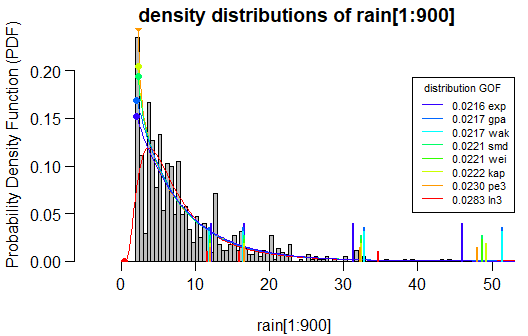The resulting parametric quantiles can be obtained with dlf$quant # distLquantile output if returnlist=FALSE (the default)
##                             80%      90%      99%    99.9%       RMSE
## exp                    12.19298 16.62461 31.34614 46.06767 0.02164835
## gpa                    11.97091 16.50472 32.84404 51.34656 0.02168117
## wak                    11.97091 16.50472 32.84404 51.34656 0.02168117
## smd                    12.04967 16.63556 32.42915 48.76870 0.02214700
## wei                    12.04978 16.63573 32.42858 48.76536 0.02214826
## kap                    12.04898 16.61889 32.44755 49.19788 0.02220832
## pe3                    12.09036 16.68269 32.23317 47.98165 0.02296758
## ln3                    11.59409 15.99297 34.69355 61.45089 0.02827637
## gno                    11.59409 15.99297 34.69355 61.45089 0.02827637
## gev                    11.33374 15.51285 35.79670 73.36759 0.03553238
## glo                    11.13388 15.09647 36.46785 84.20659 0.04120231
## gam                    12.51191 16.37376 28.30300 39.62101 0.04528978
## pdq3                   10.90010 15.35547 38.30254 66.78911 0.05103803
## lap                    10.45717 13.48705 23.55212 33.61719 0.05423815
## gum                    12.55209 16.01300 26.85001 37.49019 0.06547828
## ray                    13.00613 16.07012 23.81091 29.75062 0.07665492
## nor                    13.06524 15.55792 21.47781 25.80604 0.10397763
## rice                   11.87654 14.20564 20.08981 24.60489 0.10510552
## pdq4                   12.63012 15.26973 23.38432 31.32955 0.11079997
## revgum                 13.15337 14.80512 18.00186 19.87183 0.14317497
## empirical              12.32000 16.69000 32.19900 44.19623         NA
## quantileMean           12.27056 16.60778 32.10953 43.96441         NA
## weighted1              11.89998 15.96687 31.17152 50.27846         NA
## weighted2              11.87402 16.00728 31.54542 51.14368         NA
## weighted3              11.89893 16.38415 33.13001 53.67703         NA
## weightedc                   NaN      NaN      NaN      NaN         NA
## GPD_LMO_lmomco         12.23816 16.77987 33.03736 51.25944 0.04047069
## GPD_LMO_extRemes       12.20501 16.76039 33.25968 52.08538 0.04106371
## GPD_PWM_evir           11.95957 16.50125 32.92754 51.62957 0.04118047
## GPD_PWM_fExtremes      12.20727 16.75881 33.22079 51.96344 0.04118047
## GPD_MLE_extRemes       12.24647 16.73211 32.56139 49.92311 0.04324358
## GPD_MLE_ismev          12.24349 16.72908 32.56424 49.94252 0.04316878
## GPD_MLE_evd            12.24646 16.73210 32.56144 49.92330 0.04324322
## GPD_MLE_Renext_Renouv  12.24646 16.73210 32.56144 49.92331 0.04324323
## GPD_MLE_evir           12.00343 16.48306 32.28994 49.62508 0.04329409
## GPD_MLE_fExtremes      12.24906 16.73561 32.56691 49.92882 0.04329409
## GPD_GML_extRemes       12.27932 16.68502 31.80228 47.68903 0.04546585
## GPD_MLE_Renext_2par    16.22655 21.07322 33.40366 41.50241 0.14438208
## GPD_BAY_extRemes             NA       NA       NA       NA         NA
## n_full                900.00000       NA       NA       NA         NA
## n                     900.00000       NA       NA       NA         NA
## threshold               2.30000       NA       NA       NA         NA
• The first 17 rows for each distribution function are sorted by their goodness of fit (see below). They each yield different results, epecially for very high quantile probailities (values that are exceeded very rarely). Note that the deviation from each other increases for badly fitted distributions.
• The row quantileMean is an average of R’s 9 methods implemented in stats::quantile to determine empirical quantiles (order based statistic, keyword plotting positions).
• The rows GPD_* are the General Pareto Distribution quantiles, as estimated by a range of different R packages and methods (specified in the row names), computed by q_gpd. More on that in the next section GPD.
• The rows weighted* are averages of the quantiles estimated from the distribution functions, weighted by their goodness of fit (RMSE ecdf / cdf) in three default (and a custom) weighting schemes (see next section).

TOC

## Goodness of Fit

plotLweights(dlf, legargs=list(cex=0.8, bg="transparent") )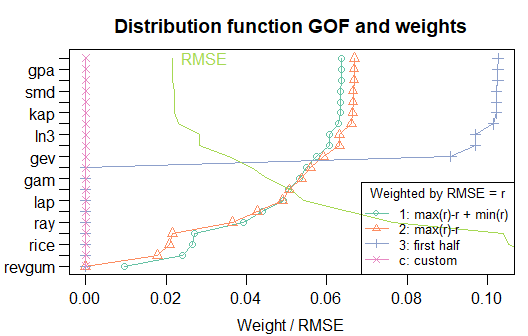The way RMSE (as a measure of GOF) is computed can be visualized nicely with a smaller dataset:

data("annMax") # annual discharge maxima in the extremeStat package itself
sel <- c("wak","lap","revgum")
dlf <- distLfit(annMax, sel=sel, quiet=TRUE)
col <- plotLfit(dlf, cdf=TRUE, sel=sel)$distcol x <- sort(annMax) for(d in 3:1) segments(x0=x, y0=lmomco::plmomco(x, dlf$parameter[[sel[d]]]),
y1=ecdf(x)(x), col=col[d])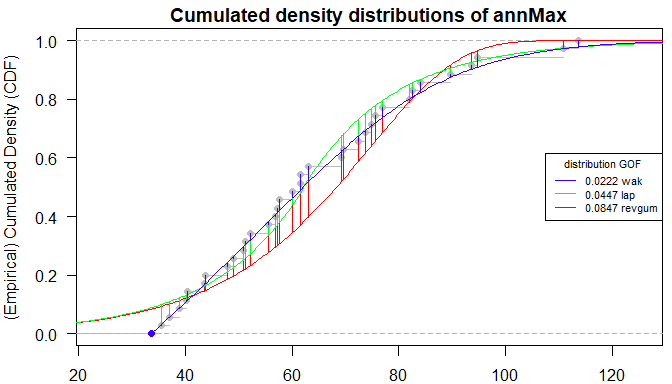The vertical bars mark the deviance of the distribution CDF from the ECDF. They are aggregated by ?rmse to mark distribution goodness of fit (GOF).

## POT, GPD

The General Pareto Distribution (‘GPD’, or ‘gpa’ in the package lmomco) is often used to obtain parametric quantile values because of the Pickands-Balkema-DeHaan theorem. It states that the tails of many (empirical) distributions converge to the GPD if a Peak-Over-Threshold (POT) method is used, i.e. the distribution is fitted only to the largest values of a sample. The resulting percentiles can be called censored or truncated quantiles.

This package is based on the philosophy that, in order to compare parametric with empirical quantiles, the threshold must be at some percentage of the full sample. That way, the probabilities given to the quantile functions can be updated.
For example, if the censored Q0.99 ( p = 0.99 ) is to be computed from the top 20 % of the full dataset (the truncated proportion is 80%: t = 0.8 ), then Q0.95 ( p2 = 0.95 ) of the truncated sample must be used. The probability adjustment for censored quantiles with truncation percentage t happens with the equation $p2 = \frac{p-t}{1-t}$
derived from

$\frac{1-p}{1-t} = \frac{1-p2}{1-0}$ as visualized along a probability line: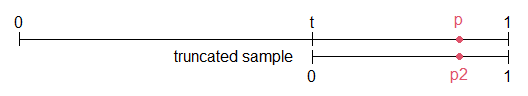In distLquantile, you can set the proportion of data discarded with the argument truncate (= t above) :

d <- distLquantile(rain, truncate=0.9, probs=0.999, quiet=TRUE, list=TRUE)
plotLquantile(d, breaks=50)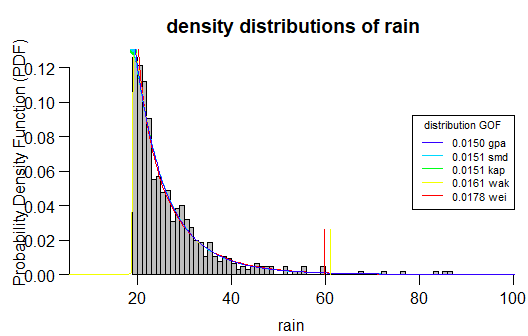TOC

## Truncation effect

To examine the effect of the truncation percentage, we can compute the quantiles for different cutoff percentages. This computes for a few minutes, so the code is not performed upon vignette creation. The result is loaded instead.

canload <- suppressWarnings(try(load("qq.Rdata"), silent = TRUE))
tt <- c(seq(0,0.5,0.1), seq(0.55,0.99,len=50))
if(interactive()) lapply <- pbapply::pblapply # for progress bars
qq <- lapply(tt, function(t)
distLquantile(rain, truncate=t, probs=0.999, quiet=TRUE, gpd=FALSE))
dlf00 <- plotLfit(distLfit(rain), nbest=17)
dlf99 <- plotLfit(distLfit(rain, tr=0.99), nbest=17)
save(tt,qq,dlf99,dlf00, file="qq.Rdata")
}

We can visualize the truncation dependency with

load("qq.Rdata")
par(mar=c(3,2.8,2.2,0.4), mgp=c(1.8,0.5,0))
plot(tt,tt, type="n", xlab="truncation proportion", ylab="Quantile estimate",
main="truncation effect for 6k values of rain", ylim=c(22,90), las=1,
xlim=0:1, xaxs="i", xaxt="n") ;  axis(1, at=0:5*0.2, labels=c("0",1:4*0.2,"1"))
dn <- dlf00$distnames ; names(dlf00$distcols) <- dn
for(d in dn) lines(tt, sapply(qq, "[", d, j=1), col=dlf00$distcols[d], lwd=2) abline(h=berryFunctions::quantileMean(rain, probs=0.999), lty=3) gof <- formatC(round(dlf00$gof[dn,"RMSE"],3), format='f', digits=3)
legend("center", paste(gof,dn), col=dlf00$distcols, lty=1, bg="white", cex=0.5) text(0.02, 62, "empirical quantile (full sample)", adj=0)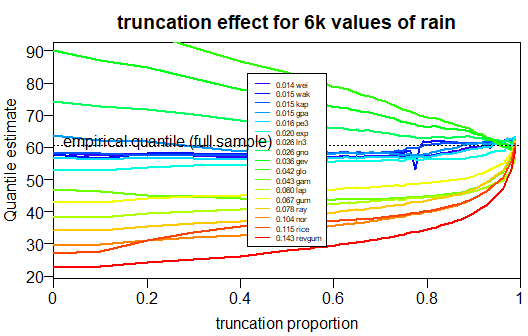The 17 different distribution quantiles (and 11 different GPD estimates, not in figure) seem to converge with increasing truncation percentage. However, at least 5 remaining values in the truncated sample are necessary to fit distributions via L-moments, so don’t truncate too much. I found a good cutoff percentage is 0.8. If you fit to the top 20% of the data, you get good results, while needing ‘only’ approximately 25 values in a sample to infer a quantile estimate. The goodness of fit also has an optimum (lowest RMSE) up to 0.8, before increasing again: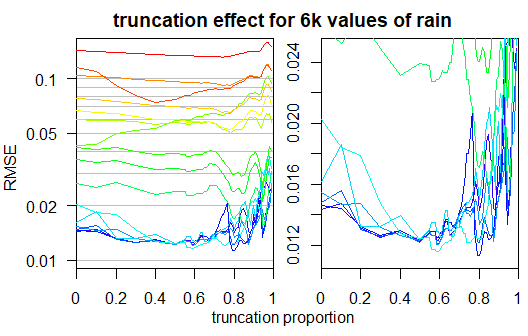TOC ## Sample size dependency One motivation behind the development of this package is the finding that high empirical quantiles depend not only on the values of a sample (as it should be), but also on the number of observations available. That is not surprising: Given a distribution of a population, small samples tend to less often include the high (and rare) values. The cool thing about parametric quantiles is that they don’t systematically underestimate the actual quantile in small samples. Here’s a quick demonstration. canload <- suppressWarnings(try(load("sq.Rdata"), silent = TRUE)) if(inherits(canload, "try-error")) { set.seed(1) ss <- c(30,50,70,100,200,300,400,500,1000) rainsamplequantile <- function() sapply(ss, function(s) distLquantile(sample(rain,s), probs=0.999, plot=F, truncate=0.8, quiet=T, sel="wak", gpd=F, weight=F)) sq <- pbapply::pbreplicate(n=100, rainsamplequantile()) save(ss,sq, file="sq.Rdata") } Loading the resulting R objects, the sample size dependency can be visualized as follows: load("sq.Rdata") par(mar=c(3,2.8,2.2,0.4), mgp=c(1.7,0.5,0)) sqs <- function(prob,row) apply(sq, 1:2, quantile, na.rm=TRUE, probs=prob)[row,] berryFunctions::ciBand(yu=sqs(0.6,1), yl=sqs(0.4,1), ym=sqs(0.5,1), x=ss, ylim=c(25,75), xlim=c(30,900), xlab="sample size", ylab="estimated 99.9% quantile", main="quantile estimations of small random samples", colm="blue") berryFunctions::ciBand(yu=sqs(0.6,2), yl=sqs(0.4,2), ym=sqs(0.5,2), x=ss, add=TRUE) abline(h=quantile(rain,0.999)) text(250, 50, "empirical", col="forestgreen") text(400, 62, "Wakeby", col="blue") text(0, 61, "'True' population value", adj=0) text(600, 40, "median and central 20% of 100 simulations")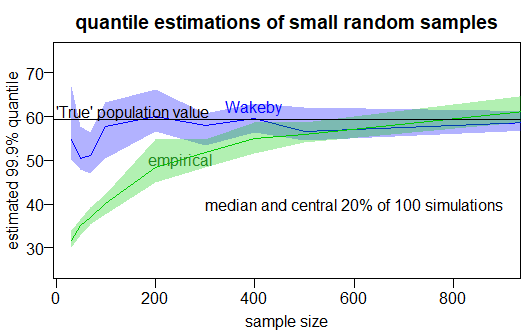TOC ## Extreme value statistics, Return Periods Once you have a quantile estimator, you can easily compute extremes (= return levels) for given return periods. A value x in a time series has a certain expected frequency to occur or be exceeded: the exceedance probability Pe. The Return Period (RP) of x can be computed as follows: $RP = \frac{1}{Pe} = \frac{1}{1-Pne}$ From that follows for the probability of non-exceedance Pne: $Pne = 1 - \frac{1}{RP}$ The Return Level RL can be computed as $RL = quantile(x, ~~ prob=Pne)$ The Return Periods are given in years, thus if you use daily values in a POT approach, you should set npy=365.24, as the formula in this package uses $returnlev = distLquantile(x, ~~ probs=1-\frac{1}{RP*npy})$ An example with actual numbers (only valid in stationary cases!!): A once-in-a-century-flood (event with a return period of 100 years) is expected to be exceeded ca. ten times in a dataset spanning a millennium. Let the associated discharge (river flow) be 5400 m^3/s. The exceedance probability of 5400 m^3/s is 1%, which means that in any given year, the probability to have a flood larger than that is 1%. The probability that the largest flood in 2017 is smaller than 5400 is thus 99 %. RP = 100, Pe=0.01, Pne=0.99. Let there be 60 years of daily discharge records. In the annual block maxima approach with 60 values, the probability passed to the quantile function to compute the 100-year flood is 0.99. In the peak over threshold approach with daily values above a threshold, it is 0.9999726. Here is an example with annual block maxima of stream discharge in Austria: dlf <- distLextreme(annMax, quiet=TRUE) plotLextreme(dlf, log=TRUE, legargs=list(cex=0.6, bg="transparent"), nbest=17)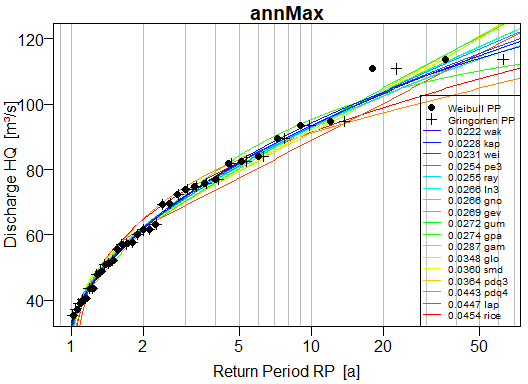dlf$returnlev[1:20,]
##            RP.2     RP.5    RP.10     RP.20     RP.50
## wak    62.06908 82.00224 93.37393 103.30175 114.81836
## kap    61.63990 82.43319 94.20990 103.80750 113.98584
## wei    61.84405 81.72957 93.39678 103.55521 115.46953
## pe3    61.86107 81.13112 92.97753 103.72004 116.87811
## ray    62.37416 81.92136 93.07332 102.63851 113.71311
## ln3    61.89473 80.85126 92.71419 103.69028 117.47486
## gno    61.89473 80.85126 92.71419 103.69028 117.47486
## gev    61.85979 80.83924 92.82171 103.89743 117.64984
## gum    61.24316 80.26879 92.86542 104.94841 120.58860
## gpa    61.36114 84.02187 95.30208 103.19519 110.11583
## gam    62.54834 81.39612 92.57994 102.53018 114.52039
## glo    62.16467 79.38862 91.10085 103.11624 120.24369
## smd    62.02142 79.68616 91.92993 104.02826 119.97815
## pdq3   61.89525 79.48144 91.89691 104.32582 120.86389
## pdq4   64.78000 83.10872 91.06007  97.39781 104.81257
## lap    62.19140 77.33774 88.79551 100.25328 115.39963
## rice   64.59196 82.14649 91.37297  99.01113 107.62436
## nor    64.78000 82.13652 91.20908  98.70136 107.13390
## exp    57.63946 78.96177 95.09148 111.22119 132.54351
## revgum 68.31684 82.45728 88.46912  92.88645  97.36604

Explore the other possibilities of the package by reading the function help files.
A good place to start is the package help:

?extremeStat

TOC

Any feedback on this package (or this vignette) is very welcome via github or !